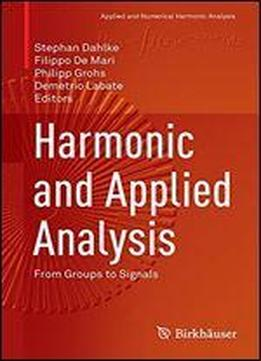# Harmonic And Applied Analysis: From Groups To Signals (applied And Numerical Harmonic Analysis) by Stephan Dahlke / 2015 / English / PDF

This contributed volume explores the connection between the theoretical aspects of harmonic analysis and the construction of advanced multiscale representations that have emerged in signal and image processing. It highlights some of the most promising mathematical developments in harmonic analysis in the last decade brought about by the interplay among different areas of abstract and applied mathematics. This intertwining of ideas is considered starting from the theory of unitary group representations and leading to the construction of very efficient schemes for the analysis of multidimensional data. After an introductory chapter surveying the scientific significance of classical and more advanced multiscale methods, chapters cover such topics as -An overview of Lie theory focused on common applications in signal analysis, including the wavelet representation of the affine group, the Schrdinger representation of the Heisenberg group, and the metaplectic representation of the symplectic group -An introduction to coorbit theory and how it can be combined with the shearlet transform to establish shearlet coorbit spaces -Microlocal properties of the shearlet transform and its ability to provide a precise geometric characterization of edges and interface boundaries in images and other multidimensional data -Mathematical techniques to construct optimal data representations for a number of signal types, with a focus on the optimal approximation of functions governed by anisotropic singularities. A unified notation is used across all of the chapters to ensure consistency of the mathematical material presented. Harmonic and Applied Analysis:From Groups to Signalsis aimed at graduate students and researchers in the areas of harmonic analysis and applied mathematics, as well as at other applied scientists interested in representations of multidimensional data. It can also be used as a textbook for graduate courses in applied harmonic analysis.

views: 379